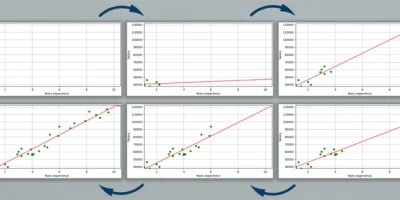## Animate Line Fitting of Simple Linear Regression using Matplotlib

Simple linear regression is a statistical method that is used to analyze the relationship between two continuous variables. During a line fitting process using linear regression, it can be useful...## Get Dataset Metadata from Kaggle using API and Python